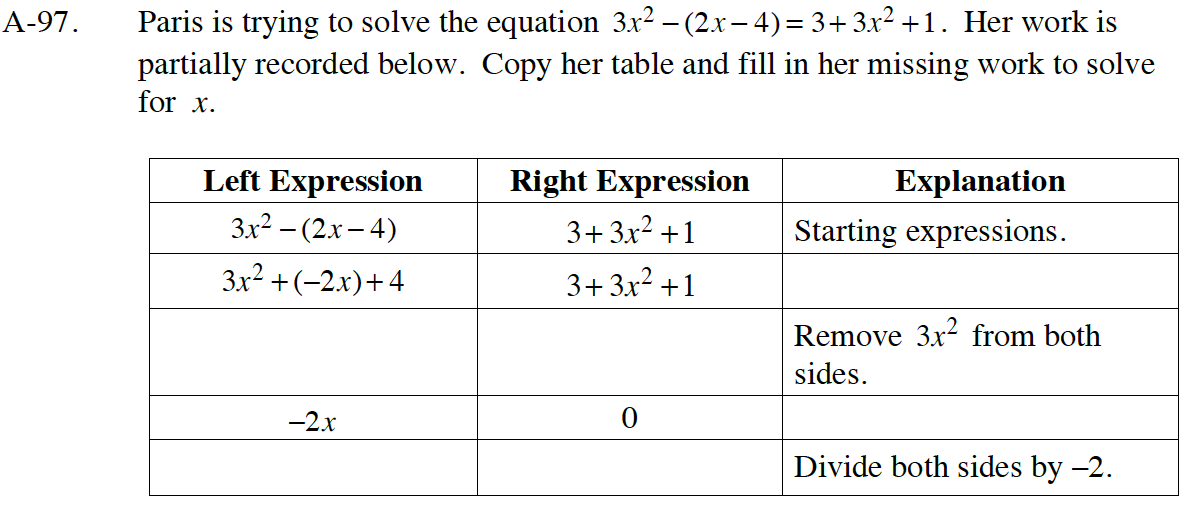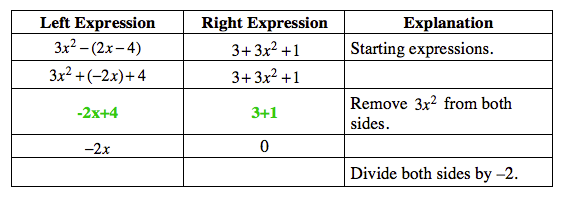Home > CC4 > Chapter A > Lesson A.1.9 > ProblemA-97

A-97.
1. Paris is trying to solve the equation 3x2 − (2x − 4) = 3 + 3x2 + 1. Her work is partially recorded below. Copy her table and fill in her missing work to solve for x. Homework Help ✎

2.  Left Expression Right Expression Explanation 3x2 − (2x − 4) 3 + 3x2 + 1 Starting expressions. 3x2 + (−2x) + 4 3 + 3x2 + 1 Remove 3x2 from both sides. −2x 0 Divide both sides by –2.Solve the parentheses on the left.Remove 4 from both sides.

x = 0